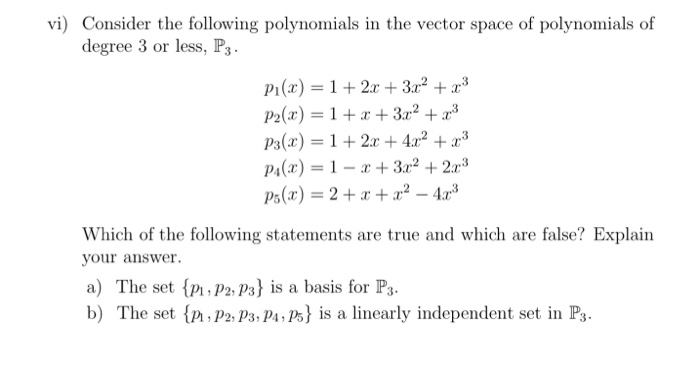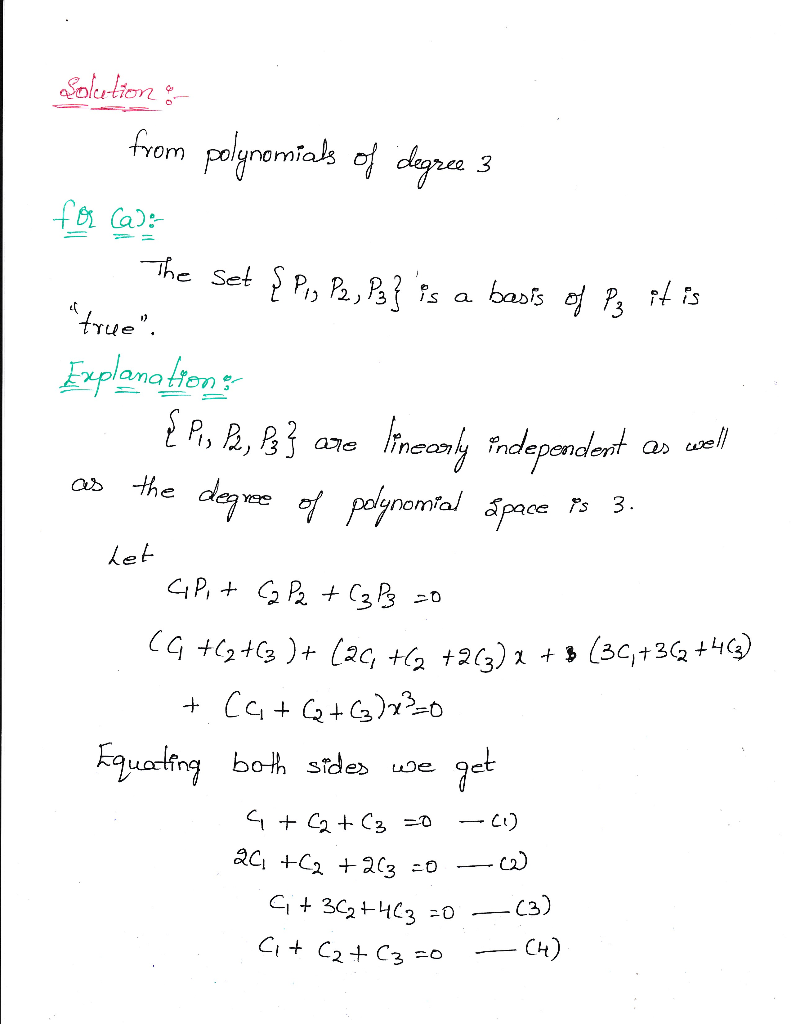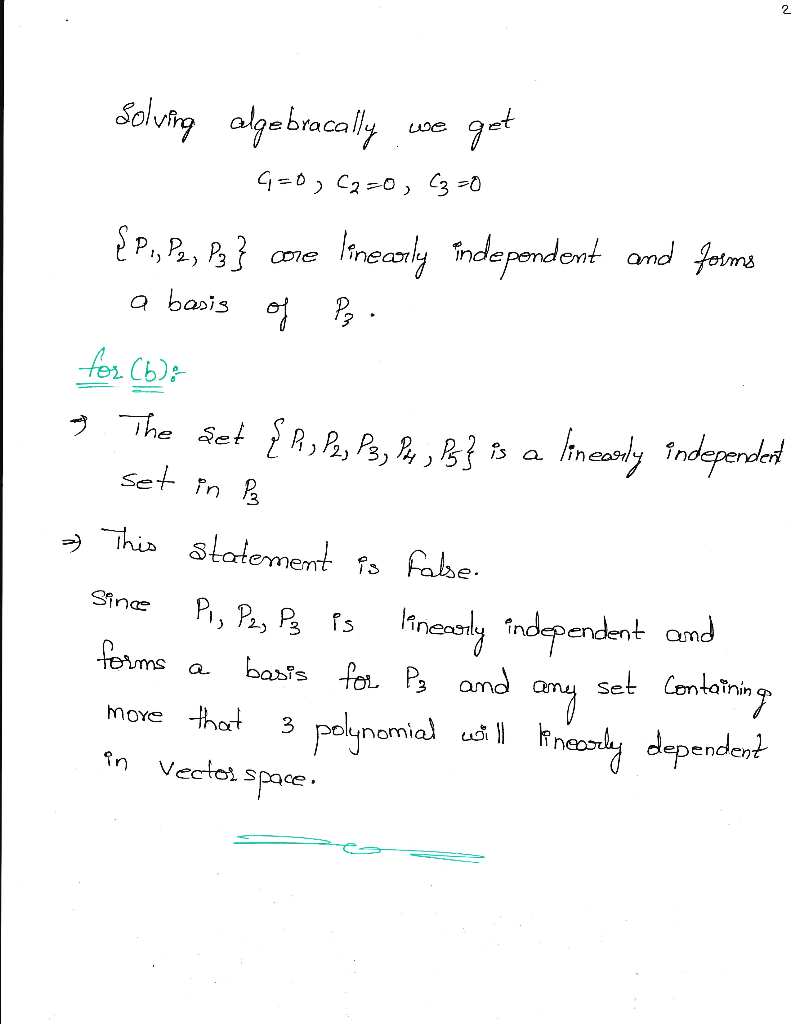Homework Help Question & Answers

# vi) Consider the following polynomials in the vector space of polynomials of degree 3 or less, P3. Pi(x) 12 +3r2 +a3 P2(x) 132 Pa(r) 1242 P4(z) = 1-r + 3r2 + 2r3 Which of the following statements...vi) Consider the following polynomials in the vector space of polynomials of degree 3 or less, P3. Pi(x) 12 +3r2 +a3 P2(x) 132 Pa(r) 1242 P4(z) = 1-r + 3r2 + 2r3 Which of the following statements are true and which are false? Explain your answer. a) The set {Pi, P2,P3} is a basis for P3. b) The set {Pi,P2, p3,P4,P5} İs a linearly independent set in P3.

Given that:##### Add Answer of: vi) Consider the following polynomials in the vector space of polynomials of degree 3 or less, P3. Pi(x) 12 +3r2 +a3 P2(x) 132 Pa(r) 1242 P4(z) = 1-r + 3r2 + 2r3 Which of the following statements...
More Homework Help Questions Additional questions in this topic.

• #### E the vector space of polynomials over R of degree less than 3. Define a quadratic form on V by a...

Need Online Homework Help?

Get FREE EXPERT Answers
WITHIN MINUTES
Related Questions